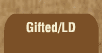Mathematics and Dyslexia

Tips for Learning the Times Tables

Tools for the Times Tables & Workbook

Home > Math > times tables practice > tens times tables

## The Tens Times Tables

For whole numbers, you can apply this rule:

When you multiply by ten
Stick a zero at the end.

At this level, you're also expected to know that in multiplication you can switch numbers back and forth; that 3 x 10 is the same thing as 10 x 3. If you don't believe this, try making 10 rows of 3 pennies and looking at it sideways -- it's 3 rows of 10 pennies.

1. 10 x 1=
2. 3 x 10=
3. 10 x 10=
4. 9 x 10=
5. 10 x 0=
6. 7 x 10=
7. 10 x 5=
8. 2 x 10=
9. 8 x 10=
10. 10 x 4=
11. 10 x 6=
12. 5 x 10=
13. 10 x 7=
14. 10 x 3=
15. Challenge yourself! 214 x 10=
16. BONUS!! 7 x 6=